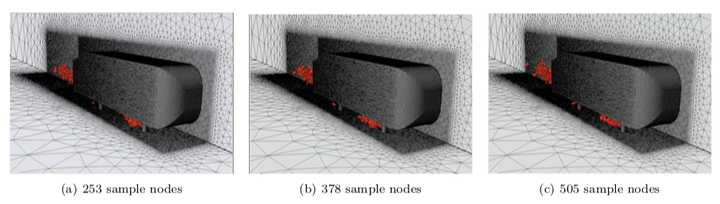# The GNAT nonlinear model reduction method and its application to fluid dynamics problemsSample meshes generated using snapshot method 1.

### Abstract

The goal of this work is to accurately evaluate large-scale, nonlinear, finite-volume-based fluid dynamics models at low computational cost. To accomplish this objective, this work employs the Gauss– Newton with approximated tensors (GNAT) nonlinear model reduction method originally presented in Ref. 1. This technique decreases the system dimension by a least-squares Petrov–Galerkin projec- tion, and decreases computational complexity by approximating the residual and Jacobian using the ‘Gappy POD’ method; the latter requires computing only a few rows of the approximated quanti- ties. This work introduces an efficient implementation of the GNAT method based on a novel “sample mesh” concept tailored for the finite volume formulation. When the reduced-order model is evaluated, this approach loads into memory only the subset of the mesh needed to sample the residual and Jaco- bian. This minimizes required computational resources, communication overhead, and computational complexity. A post-processing step that employs only the subset of the mesh needed for computing outputs is also proposed. Results obtained for a one-dimensional shock propagation problem highlight the method's capability to decrease solution times by orders of magnitude while retaining high levels of accuracy, even in predictive scenarios. The application of GNAT to a large-scale, compressible, turbulent flow problem with over 17 million unknowns illustrates the method's favorable performance compared with other nonlinear model reduction techniques (including collocation and discrete empirical interpolation approaches), and speedups exceeding 350 with errors less than 1% are observed. Finally, results show that the sample mesh enables the GNAT model to use many fewer processors compared with the full-order simulation.

Publication
AIAA Paper 2011-3112, 6th AIAA Theoretical Fluid Mechanics Conference, Honolulu, Hawaii, June 27–30, 2011